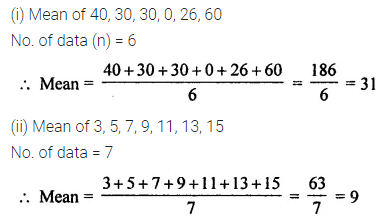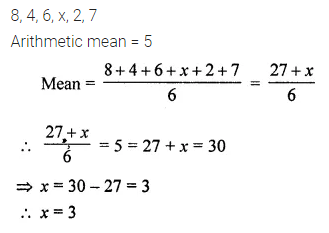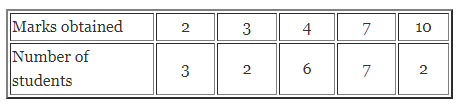# ML Aggarwal Class 7 Solutions for ICSE Maths Chapter 17 Data Handling Ex 17.2

## ML Aggarwal Class 7 Solutions for ICSE Maths Chapter 17 Data Handling Ex 17.2

Question 1.
Find the mean of the following data:
(i) 40, 30, 30, 0, 26, 60
(ii) 3, 5, 7, 9, 11, 13, 15
Solution:Question 2.
Find the mean of the first five whole numbers.
Solution:Question 3.
A batsman scored the following number of runs in six innings:
36, 35, 50, 46, 60, 55
Calculate the mean runs scored by him in an inning.
Solution:Question 4.
The enrolment in a school for six consecutive years was as follows:
1555, 1670, 1750, 2013, 2540, 2825
Find the mean enrolment of the school for this period.
Solution:Question 5.
The marks (out of 100) obtained by a group of students in a science test are:
85, 76, 90, 85, 39, 48, 56, 95, 81, 75
Find the:
(i) highest and lowest marks obtained by the students.
(ii) range of the marks obtained.
(iii) mean marks obtained by the students.
Solution:Question 6.
The heights of 10 girls were measured in cm and the results are as follows:
135, 150, 139, 128, 151, 132, 146, 149, 143, 141
(i) What is the height of the tallest girl?
(ii) What is the height of the shortest girl?
(iii) What is the mean height of the girls?
(iv) How many girls have heights more than the mean height?
Solution:Question 7.
If the arithmetic mean of 8, 4, 6, x, 2, 7 is 5, then find the value of x.
Solution:Question 8.
Find the mean of the following data:Solution: